BetMGM Make Playoffs Odds
-1000
0.1 to 1Chiefs
87.2% implied probability

No changes have been recorded yet.
-625
0.16 to 1Buccaneers
82.6% implied probability

-357
0.28 to 1Bills
74.8% implied probability

-303
0.33 to 1Ravens
71.9% implied probability

-200
0.5 to 1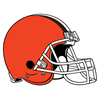Browns
63.9% implied probability

-189
0.53 to 149ers
62.5% implied probability

-182
0.55 to 1Rams
61.7% implied probability

-141
0.71 to 1Cowboys
55.7% implied probability

-135
0.74 to 1Titans
55.3% implied probability

-119
0.84 to 1Seahawks
51.6% implied probability

-105
0.95 to 1Saints
48.9% implied probability

+110
1.1 to 1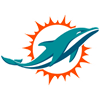Dolphins
45.3% implied probability

+110
1.1 to 1Patriots
45.3% implied probability

+110
1.1 to 1Chargers
45.3% implied probability

+120
1.2 to 1Football Team
43.4% implied probability

+130
1.3 to 1Broncos
41.8% implied probability

+140
1.4 to 1Steelers
39.9% implied probability

+150
1.5 to 1Cardinals
38.3% implied probability

+220
2.2 to 1Falcons
29.8% implied probability

+225
2.25 to 1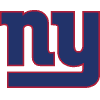Giants
29.5% implied probability

No changes have been recorded yet.
+240
2.4 to 1Panthers
28.3% implied probability

+250
2.5 to 1Eagles
27.4% implied probability

+350
3.5 to 1Jaguars
21.3% implied probability

+375
3.75 to 1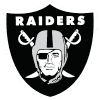Raiders
20.2% implied probability

+525
5.25 to 1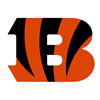Bengals
15.3% implied probability

+600
6 to 1Jets
13.7% implied probability

+900
9 to 1Texans
9.6% implied probability